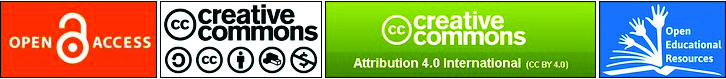### COGNITIVE MODELLING SKILLS FROM NOVICIATE TO EXPERTNESS

Ayşe Tekin Dede, Süha Yilmaz

#### Abstract

The purpose of this study is to reveal the cognitive modelling skills of 6th grade students after a long term modelling implementation. The cognitive modelling skills are regarded as understanding the problem, simplifying, mathematising, working mathematically, interpreting and validating. Seven-month modelling sequences were designed and conducted, and the first and last implementations were particularly examined in the study. The participants were four students, while the data collection tools were solution papers for two different modelling problems in the implementations and transcriptions of the video records concerning the solution and solution presentation processes. When the data were analysed through a rubric and presented descriptively, it was seen that a development was revealed in cognitive modelling skills from noviciate to expertness. In other words, the students displayed richer approaches in the context of each cognitive modelling skill in the last implementation.

Article visualizations:#### References

Biccard, P. (2010). An investigation into the development of mathematical modelling competencies of Grade 7 learners. Published Masters Dissertation, Stellenbosch University.

Blomhøj, M., & Jensen, T. H. (2003). Developing mathematical modelling competence: conceptual clarification and educational planning. Teaching Mathematics and Its Applications, 22(3), 123-139.

Blomhøj, M., & Kjeldsen, T. N. (2006). Teaching mathematical modelling through project work. Zentralblatt für Didaktik der Mathematik-ZDM, 38(2), 163-177.

Blum, W., & Leiß, D. (2007). How do students and teachers deal with modelling problems? In C. Haines, P. Galbraith, W. Blum & S. Khan (Eds.), Mathematical Modelling (ICTMA 12): Education, Engineering and Economics (pp. 222-231). Chichester: Hollywood.

Blum, W. (2011). Can modelling be taught and learnt? Some answers from empirical research. In G. Kaiser, W. Blum, R. Borromeo Ferri & G. Stillman (Eds.), Trends in Teaching and Learning of Mathematical Modelling. International Perspectives on the Teaching and Learning of Mathematical Modelling (pp. 15-30). New York: Springer.

Borromeo Ferri, R. (2006). Theoretical and empirical differentiations of phases in the modelling process. Zentralblatt für Didaktik der Mathematik-ZDM, 38(2), 86-95.

Bracke, M., & Geiger, A. (2011). Real-world modelling in regular lessons: A long-term experiment. In G. Kaiser, W. Blum, R. Borromeo Ferri & G. Stillman (Eds.), Trends in Teaching and Learning of Mathematical Modelling. International Perspectives on the Teaching and Learning of Mathematical Modelling (pp. 529-549). New York: Springer.

Bukova Güzel, E. (2011). An examination of pre-service mathematics teachers’ approaches to construct and solve mathematical modeling Problems. Teaching Mathematics and Its Applications, 30(1), 19-36.

Chan, C. M. E. (2010). Tracing primary 6 pupils’ model development within the mathematical modelling process. Journal of Mathematical Modelling and Application, 1(3), 40-57.

Chan, C. M. E., & Foong, P. Y. (2013). A Conceptual Framework for Investigating Pupils’ Model Development during the Mathematical Modelling Process. The Mathematics Educator, 13 (1), 1-29.

Grünewald, S. (2012). Acquirement of modelling competencies – First results of an Empirical comparison of the effectiveness of a holistic respectively an atomistic approach to the development of (metacognitive) modelling competencies of students. 12th International Congress on Mathematical Education, 8 July-15 July 2012, COEX, Seoul, Korea.

Grünewald, S. (2013). The development of modelling competencies by year 9 students: Effects of a modelling project. In G. A. Stillman, G. Kaiser, W. Blum & J. P. Brown (Eds.), Teaching Mathematical Modelling: Connecting to Research and Practice. International Perspectives on the Teaching and Learning of Mathematical Modelling (pp. 185-194). New York: Springer.

Ji, X. (2012). A quasi-experimental study of high school students’ mathematics modelling competence. 12th International Congress on Mathematical Education, 8 July-15 July 2012, COEX, Seoul, Korea.

Kaiser, G., & Maaß, K. (2007). Modelling in Lower secondary mathematics classroom – problems and opportunities. In W. Blum, P. L. Galbraith, H. W. Henn & M. Niss (Eds.), Modelling and Applications in Mathematics Education: The 14th ICMI Study (pp 99-108). Springer: New York.

Kaiser, G., & Schwarz, B. (2006). Mathematical modelling as bridge between school and university. Zentralblatt für Didaktik der Mathematik-ZDM, 38, 196-208.

Kaiser, G. (2007). Modelling and modelling competencies in school. In C. Haines, P. Galbraith, W. Blum & S. Khan (Eds.), Mathematical modelling (ICTMA 12): Education, engineering and economics: proceedings from the twelfth International Conference on the Teaching of Mathematical Modelling and Applications (pp. 110-119). Chichester: Horwood.

Krippendorff, K. (1980). Content Analysis: An Introduction to its Methodology. Beverly Hills, CA: Sage Publications.

Lesh, R., & Caylor, B. (2007). Introduction to special issue: modeling as application versus modeling as a way to create mathematics. International Journal of Computers for Mathematical Learning, 12(3), 173-194.

Maaß, K. (2005). Barriers and opportunities for the integration of modelling in mathematics classes: Results of an empirical study. Teaching Mathematics and Its Applications, 24(2-3), 61-74.

Maaß, K. (2006). What are modelling competencies? Zentralblatt für Didaktik der Mathematik-ZDM, 38(2), 113-142.

Maaß, K., & Gurlitt, J. (2011). Designing a teacher questionnaire to evaluate professional development in modelling. In V. Durand-Guerrier, S. Soury-Lavergne & F. Arzarello (Eds.), Proceedings of the 6th Congress of the European Society for Research in Mathematics Education CERME 6 (pp. 2056-2065). France: Lyon.

Maaß, K., & Mischo, C. (2011). Implementing modelling into day-to-day teaching practice-The project STRATUM and its framework. Journal Für Mathematik-Didaktik, 32(1), 103-131.

Miles, M. B., & Huberman, M. A. (1994). Qualitative Analysis: An Expanded Sourcebook. Thousand Oaks, CA: Sage.

Ministry of National Education [MNE], (2012a). Ortaokul ve İmam Hatip Ortaokulu Matematik Uygulamaları I. Dönem Öğretmenler İçin Öğretim Materyali. Ankara.

Ministry of National Education [MNE], (2012b). Ortaokul ve İmam Hatip Ortaokulu Matematik Uygulamaları II. Dönem Öğretmenler İçin Öğretim Materyali. Ankara.

Schukajlow, S., Leiss, D., Pekrun, R., Blum, W., Müller, M., & Messner, R. (2012). Teaching methods for modelling problems and students’ task-specific enjoyment, value, interest and self-efficacy expectations. Educational Studies in Mathematics, 79, 215-237.

Weber, R. P. (1985). Basic Content Analysis, Quantitative Applications in the Social Sciences. Beverly Hills, CA: Sage Publications.

DOI: http://dx.doi.org/10.46827/ejes.v0i0.334

### Refbacks

• There are currently no refbacks.

Copyright (c) 2018 Ayşe Tekin Dede, Süha Yilmaz